# Know Your OpenGL Tessellation Spacing ModesIn OpenGL 4 (as well as in Direct3D 11), the tessellation levels are specified with floating points numbers. For example, if the tessellation level is 3.0 (for both outer and inner levels), a edge will be divided in three parts (or three segments). But in how many segments the same edge will be subdivided if the tessellation level is 3.25? And 3.75?

The answer is given by the tessellation spacing (or fractionning in Direct3D 11). There are 3 spacing modes in OpenGL:

• equal_spacing: the tessellation level is clamped to the range [1, 64] and is rounded up to the nearest integer n, and the corresponding edge is divided into n segments. Example: a level of 3.75 will be rounded up to 4 and the edge will be divided in 4 identical segments.
• fractional_odd_spacing: the tessellation level is clamped to the range [1, 63] and then rounded up to the nearest odd integer n. Example: a level of 3.75 will be rounded up to 5 and the edge will be divided in 5 segments.
• fractional_even_spacing: the tessellation level is clamped to the range [1, 63] and then rounded up to the nearest even integer n. Example: a level of 3.75 will be rounded up to 4 and the edge will be divided in 4 segments.

I said that equal_spacing divides an edge in n identical segments. This is not the case with fractional_odd_spacing and fractional_even_spacing. With these modes, segments can be identical or not depending on the tessellation level. For example, with fractional_odd_spacing, a tessellation level of 7.0 will produce 7 identical segments while a tessellation level of 8.0 will produce 9 different segments (7 identical + 2 smaller ones).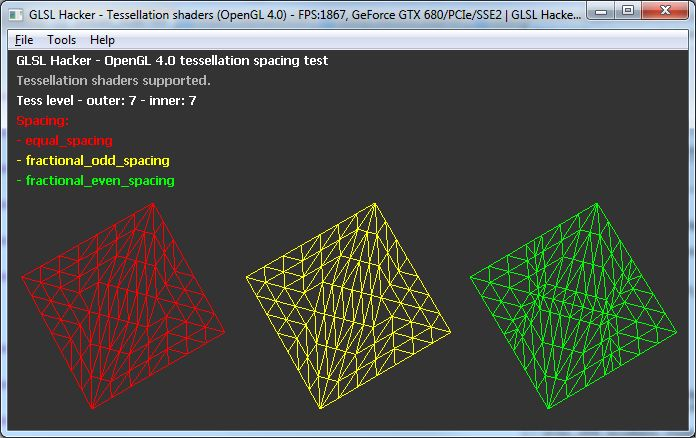Tessellation level of 7 leads to 7 identical segments for fractional_odd_spacing (yellow)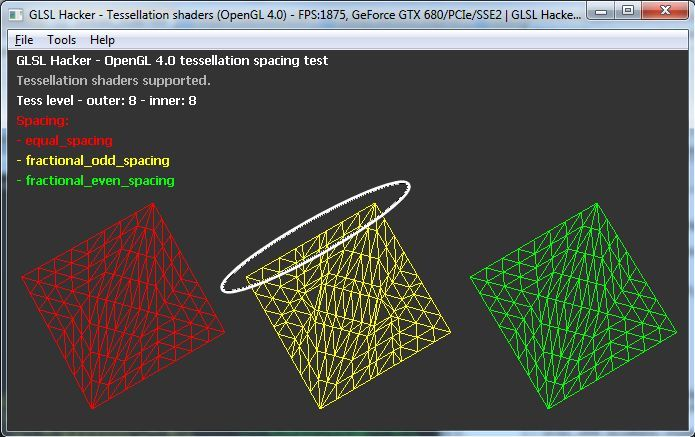Tessellation level of 8 leads to 9 different segments for fractional_odd_spacing (yellow)

The tessellation spacing modes are defined in the tessellation evaluation shader with the following syntax in the case of a triangle patch:

```layout(triangles, equal_spacing) in;
```

or

```layout(triangles, fractional_even_spacing) in;
```

or

```layout(triangles, fractional_odd_spacing) in;
```

Why do we need floating point tessellation level since the hardware does not render partial triangles? An answer is that floating point tessellation levels combined with fractional_even_spacing and fractional_odd_spacing allow smooth transition between different levels of tessellation (useful for LOD algorithms). Look at the pictures below where the tessellation level goes from 3.0 to 5.0 by step of 0.25: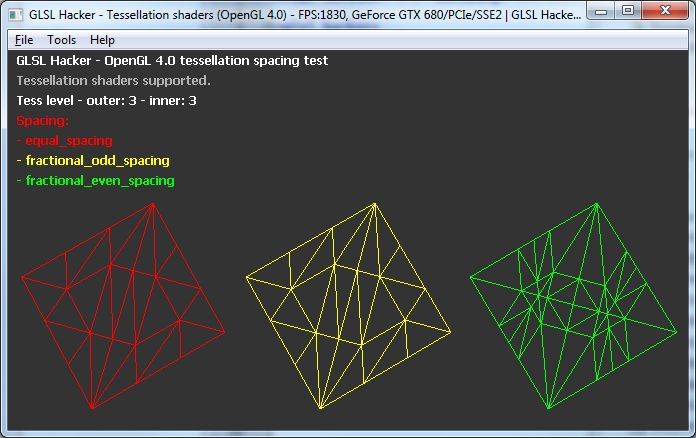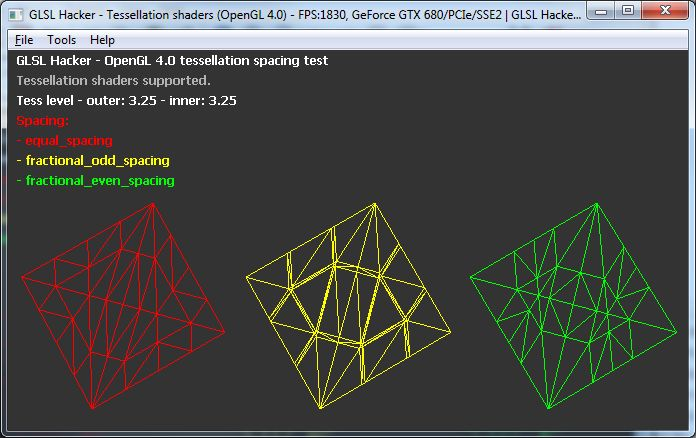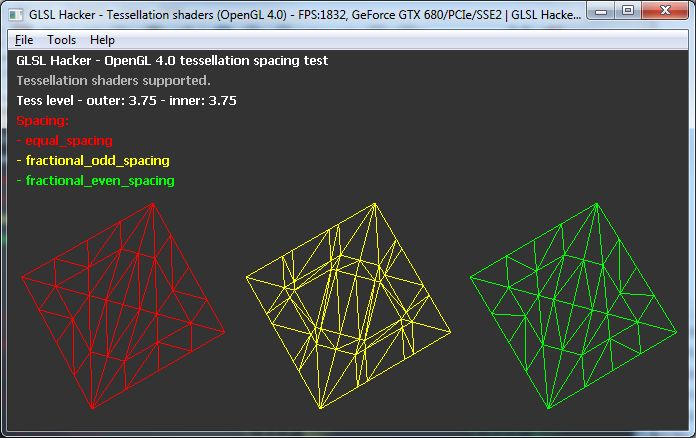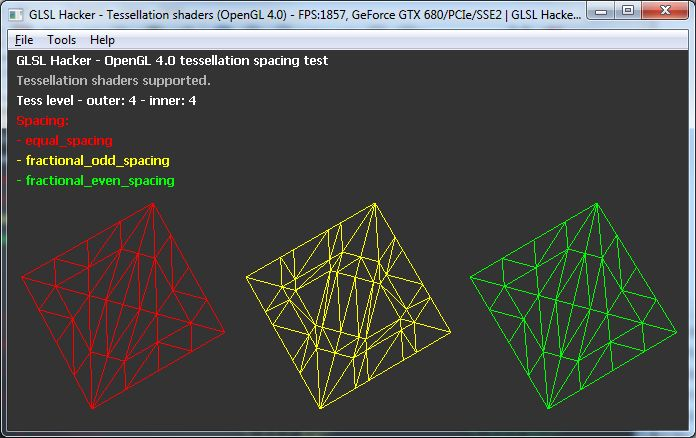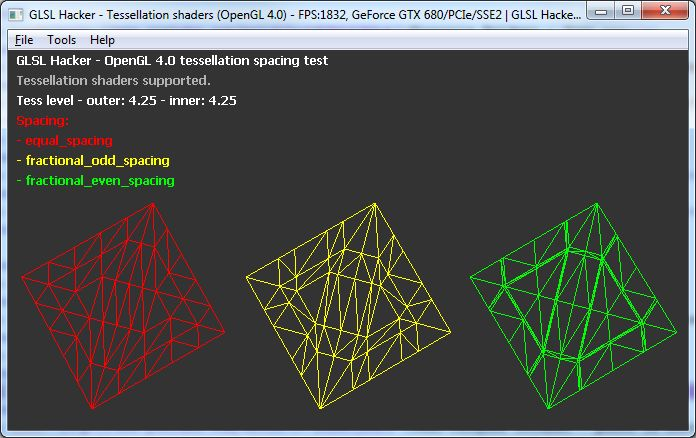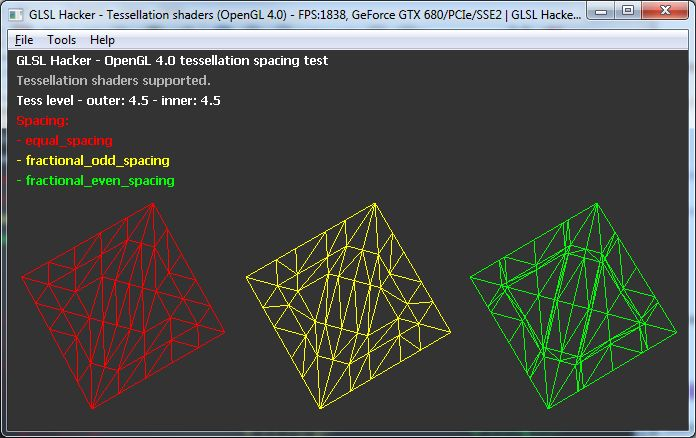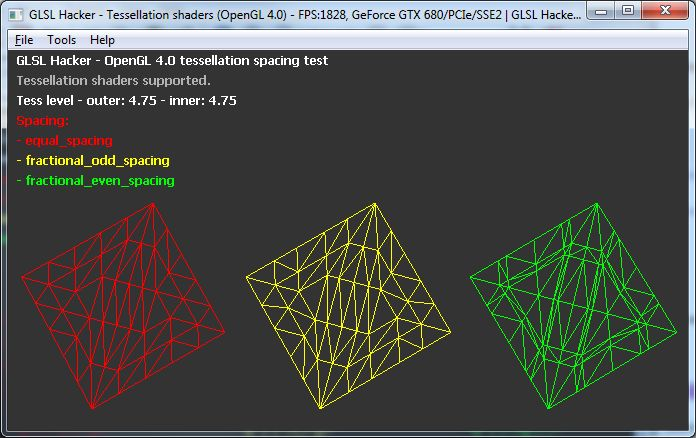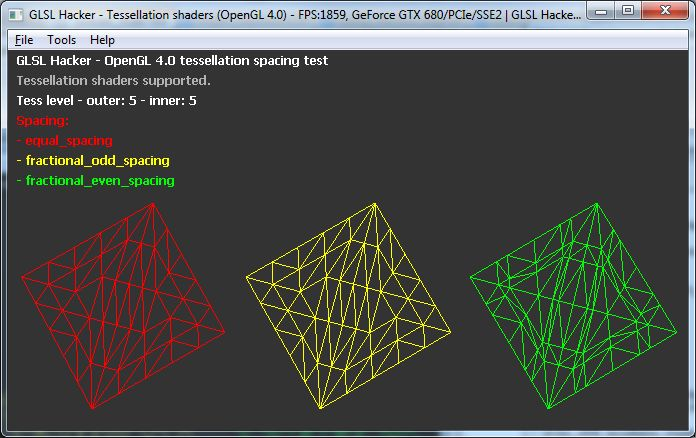You can play with the demo and live-code the tessellation shaders with GLSL Hacker (Windows, Linux and OSX). You will find the demo in the GLSL_Tessellation_Spacing/ folder of GLSL Hacker code samples pack (Note: this demo can run on Windows and Linux only).

To end up the article, here are Direct3D 11 tessellation partitioning modes:

• integer: same mode than OpenGL equal_spacing
• fractional_even: same mode than OpenGL fractional_even_spacing
• fractional_odd: same mode than OpenGL fractional_odd_spacing
• pow2: not available in OpenGL. pow2 rounds up to the next 2n integer value, so an edge can be divided in 1, 2, 4, 8, 16, 32 or 64 segments.

References

## One thought on “Know Your OpenGL Tessellation Spacing Modes”

1.zqueezy

just as a sidenote. when using triangles, it’s aweful to map fractional and also even tessellation factors to dyadic subdivision patterns.